# Code Generation

## 指令选择

### 树型 Tree pattern

• 定义：一条机器指令表示的 IR 树的一段树枝（fragment），称为树型（tree pattern）
• 常见转化规则如下：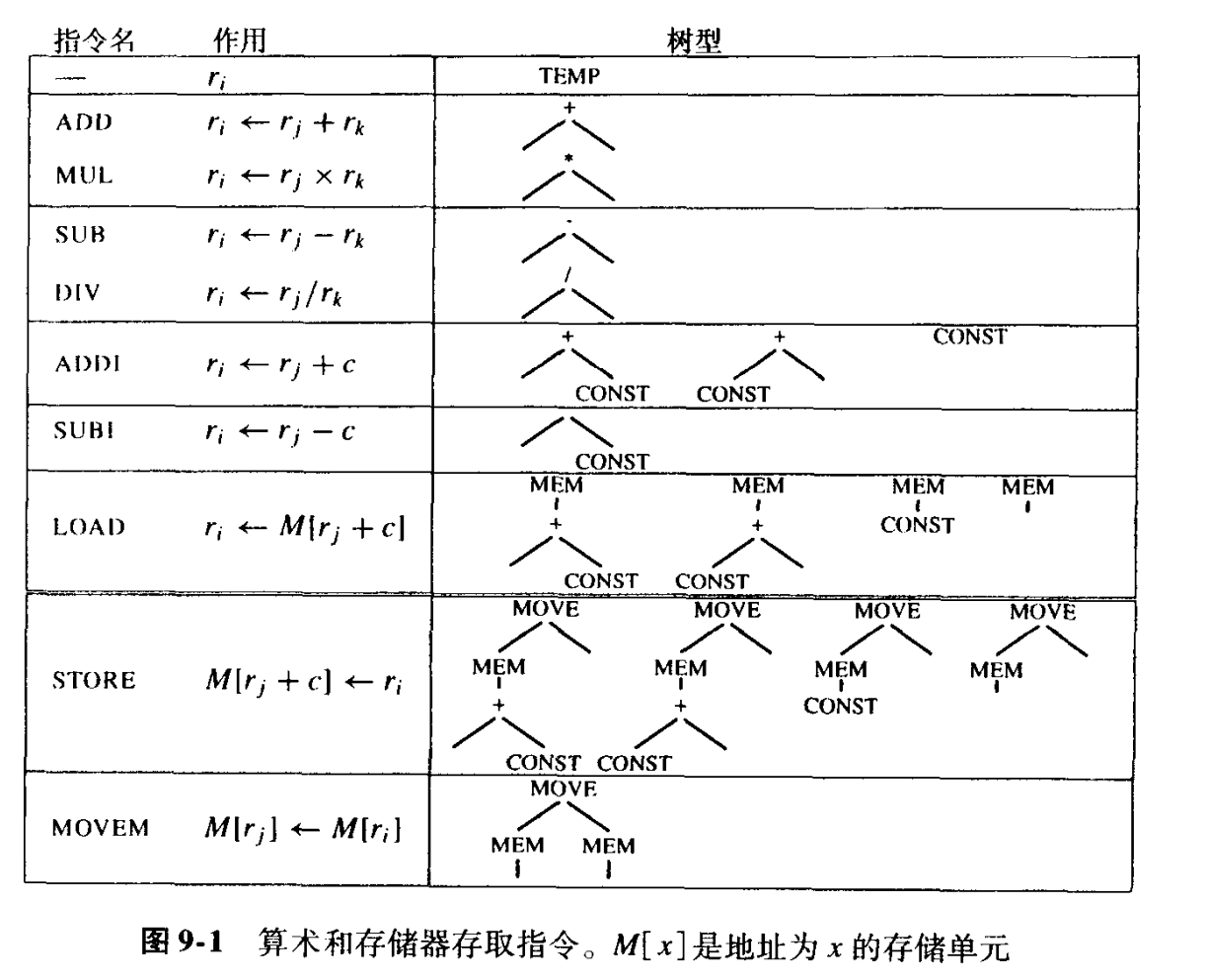### 最佳覆盖与最优覆盖

• 最优覆盖 optimum tiling：瓦片的代价之和为最小的覆盖
• 最佳覆盖 optimal tiling：不存在两个相邻的瓦片能连接成一个代价更小的瓦片
• 最优覆盖同时也是最佳的；反之不然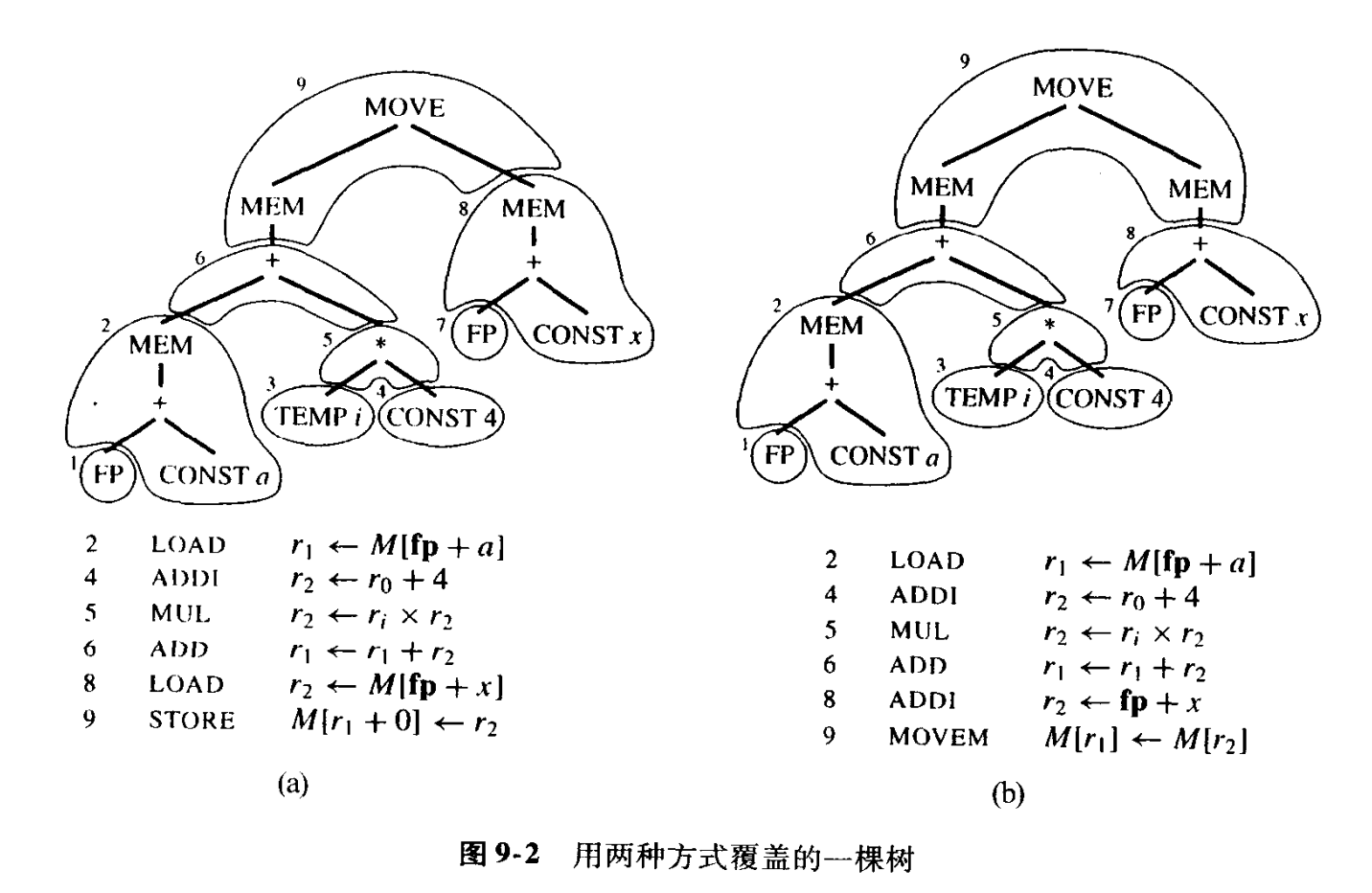### Maximal Munch 算法

• Maximal Munch 算法能够实现最佳覆盖
• Maximal Munch 算法流程
• 从根节点开始，寻找最大的瓦片，以覆盖根节点
• 对剩下的若干子树重复应用相同的算法

### 动态规划算法

• 动态规划算法能够实现最优覆盖

## 活跃分析

### 控制流图

• 定义
• 程序中的每条语句都是流图的一个结点
• 如果语句 x 之后跟随着语句 y，则会有一条从 x 到 y 的边
• 例子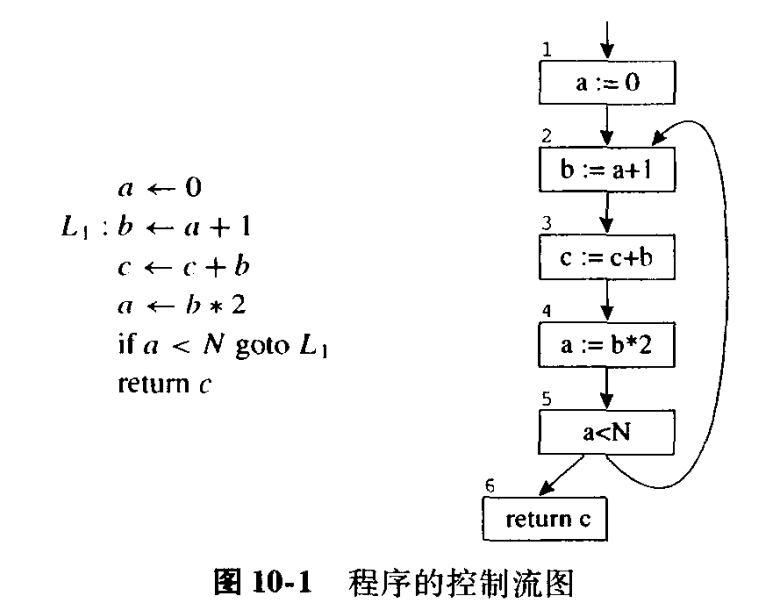### 数据流方程

#### 流图术语

• 出边 out-edge：流图的每个结点引向后继结点的边
• 入边 in_edge：流图的每个结点引向前驱结点的边
• pred[n] 是结点 n 所有前驱结点的集合
• succ[n] 是结点 n 所有后继结点的集合
• 定值 define：被赋值的变量
• 使用 use：用于赋值的变量
• 入口活跃 live-in：一个变量在一个结点的所有入边均是活跃的
• 出口活跃 live-out：一个变量在一个结点的所有出边均是活跃的
• 基本块：对于只有一个前驱和一个后继的结点，与它们的前驱结点和后驱结点合并形成基本块

#### 活跃性计算

• 如果一个变量属于 use[n]，那么它在结点 n 是入口活跃的
• 如果一个变量在结点 n 是入口活跃的，那么它在所有属于 pred[n] 的结点 m 中都是出口活跃的
• 如果一个变量在结点 n 是出口活跃的，而且不属于 def[n]，则该变量在结点 n 是入口活跃的
bool changed = false;
do {
changed = false;
// 如果一个变量属于 use[n]，那么它在结点 n 是入口活跃的
for (int i = 0; i < n; ++i) {
for (auto x: use[i]) {
changed |= PushLiveIn(i, x);
}
}
// 如果一个变量在结点 n 是入口活跃的，那么它在所有属于 pred[n] 结点 m 中都是出口活跃的
for (int i = 0; i < n; ++i) {
for (auto x: pred[i]) {
for (auto y: live_in[i]) {
changed |= PushLiveOut(x, y);
}
}
}
// 如果一个变量在结点 n 是出口活跃的，而且不属于 def[n]，则该变量在结点 n 是入口活跃的
for (int i = 0; i < n; ++i) {
for (auto x: live_out[i]) {
if (find(def[i].begin(), def[i].end(), x) == def[i].end()) {
changed |= PushLiveIn(i, x);
}
}
}
} while(changed);


#### 冲突图

• 冲突 interference：阻止将 a 和 b 分配到同一个寄存器的条件
• 冲突的几种情况
• 当 a 和 b 在程序中的同一点均活跃时，活跃范围重叠，存在冲突
• 不能对寄存器 ${r}_{1}$ 进行寻址的指令生成 a 时，a 和 ${r}_{1}$ 存在冲突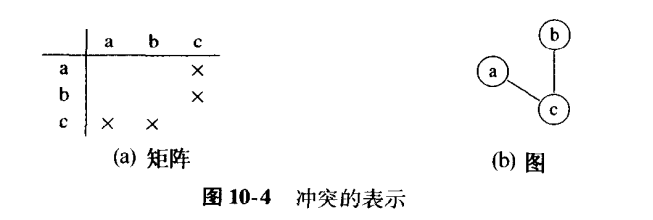## 寄存器分配

Abstract

### 简化着色问题

• 寄存器分配是一个 NP 完全问题。对于图着色问题，存在着一种能给出较好结果的线性时间近似算法，它由四个主要的处理阶段组成：构造、简化、溢出和选择。

#### 处理阶段

• 构造 build：构造冲突图
• 简化 simplify：从图中删除结点
• 算法
• 重复地删除度数小于 $K$ 的结点，并将它压入栈中
• 原理
• 假设图 $G$ 有一个结点 $m$，它的邻结点个数小于 $K$，其中 $K$ 是寄存器的个数
• ${G}^{\prime }=G-\left\{m\right\}$，若 ${G}^{\prime }$ 能够用 $K$ 色着色，那么 $G$ 也可以
• 溢出 spill
• 算法
• 对只包含高度数（significant degree）结点（度数 >= K）的图 $G$ 进行处理
• 选择图 $G$ 中的随机一个结点，将其删除并压入栈中，继续进行简化处理
• 原理
• 乐观估计结点会在将来被弹出时不会存在冲突
• 若存在冲突，会在「重新开始」步骤中解决
• 选择 select：将颜色指派给图中的结点将颜色指派给图中的结点
• 算法
• 从一个空的图开始，重复地将栈顶结点添加到图中重建原来的冲突图
• 当往图中添加一个简化阶段删除的结点时，一定会有一种它可以使用的颜色
• 当往图中添加一个溢出阶段删除的结点时，有一定概率没有可使用的颜色
• 重新开始 start over
• 算法
• 如果选择阶段不能为某个结点找到颜色，则进行改写
• 每次使用这些结点时需要先从存储器读出，使用后存回存储器
• 往程序中添加对应指令后，溢出的结点转变为几个具有较小活跃范围的结点
• 新产生的结点仍可能存在冲突，在改写后需要从头开始进行寄存器分配
• 反复迭代，直至没有溢出成功简化为止
• 原理
• 实际情况中，迭代的次数往往只有一两次

### 合并

Briggs 合并策略

George 合并策略

#### 处理阶段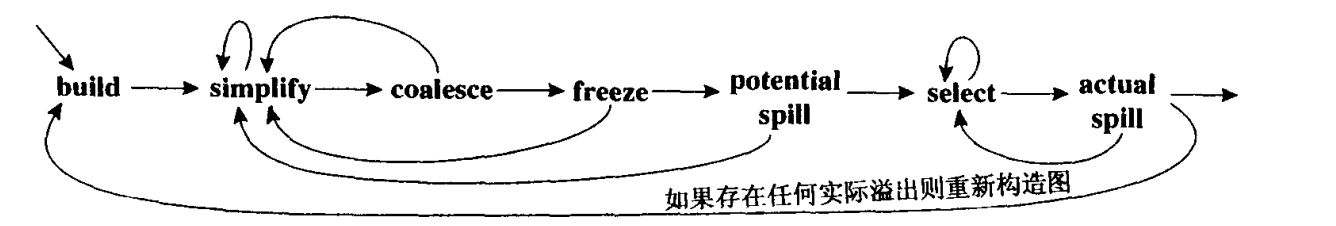• 构造：构造冲突图，将结点分类为 传送有关 的或 传送无关
• 简化：重复地删除度数小于 $K$ 的且与 传送无关 的结点，并将它压入栈中
• 合并 coalesce：对简化阶段得到的简化图施行安全合并
• 冻结 freeze
• 如果简化和合并都不能再进行，寻找一个度数较低的传送有关的结点
• 冻结这个结点所关联的那些 传送指令，将它 视作传送无关
• 重新开始简化和合并阶段
• 溢出：选择一个潜在可能溢出的高度数结点并将它压入栈
• 选择：弹出整个栈并指派颜色